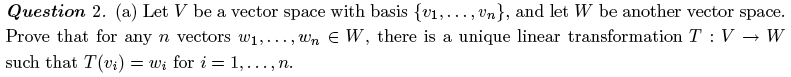# linear transformation

## Recommended Posts

hey , i'm having a bit of trouble with this linear transformation problem. i am not really sure what or how they want me to show?so yeah, does anyone have some suggestions or advice?

Cheers

Sarah##### Share on other sites

ok what i have gotten so far is...

let $v\epsilon V$

$that \ is \ v = a_1v_1+...+a_nv_n$

so

$T(v) = T(a_1v_1 + ... + a_nv_n) = T(a_1v_1) + ... + T(a_nv_n)$

$= a_1T(v_1) + ... + a_nT(v_n)$

##### Share on other sites

Please don't take this as rude, but what is yor thinking behind that step? Mightn't it be more helpful to think about how the w_i relate to the v_j?

##### Share on other sites

its fine, not taken as rude at all$v_j$ ??

##### Share on other sites

i, j, they're just dummy indieces, i could have used, s,t,r, or "squirrel", you get that it doesn't matter?

##### Share on other sites

hehe squirrel, yep i get what you mean##### Share on other sites

ok umm so how about i start by well... lol i am not really sure how to start a problem like this, never really done one like it before. how can you show that there is a unique linear transformation? i dunno :S

##### Share on other sites

Construct one that does it and the uniqueness follows (a liner map is uniquely determined by where it sends a basis and specifying the action on the basis uniquely determines the map obviously). do you understand the basic method of constructing linear maps from where they send the basis vecotrs? eg in R^2 if e and f are the basis and I tell you that e maps to 2e+f and f maps to e+2f can you write out the matrix? (you can if you just think about matrix multiplication. where does the 2x2 matrix ith entries a,b in the top row and c,d in the bottom row send the vector (0,1)^t (ie the column vector with 1 at the top and 0 at the bottom) in terms of a,b,c, adn d?

##### Share on other sites

hmmm....yeah i dunno, i can't find anything in my text book that seems to help me, there is stuff about linear transformations, but sort of dunno i don't see how to apply it to this particular question :S

##### Share on other sites

"a liner map is uniquely determined by where it sends a basis and specifying the action on the basis uniquely determines the map obviously"

is that a thm. of some kind, because i havent seen it in my textbook yet. although i can see that it makes sense.

##### Share on other sites

oh ok i think i see....

a linear transformation maps one vector space into another, right?

so if T:E --> W is a linear transformation

the range of the transformation is depedent on the vectors in V, and if you have a basis for E, then you effectively have all basis for the range of T, as a linear transformation is defined by

T(u+v) = T(u) + T(v)

and

T(cu) = cT(u)

is what i just said correct?....or relevant?Thanks

Sarah##### Share on other sites

yes, that is the idea of it, if you know where it sends the basis you know ehrere it sends every vector.

##### Share on other sites

ok heres my attempt at what i think the proof to problem should look like (tell me what you think!)

ok here goes:

-----------------------------------------------------------------------------------------

-----------------------------------------------------------------------------------------

Define T to be

$T( \sum_{i=1}^n(a_iv_i)) = \sum_{i=1}^n(a_iw_i)$

Since T is defined as transforming the basis vectors of the vector space V, then it is well defined.

Now take

$v, p \in V$

and

$c,d \in \mathbb{R}$ (i.e. c,d scalars)

So

$v = \sum_{i=1}^n(a_iv_i)$

$p = \sum_{i=1}^n(b_iv_i)$

$(cv + cp) = c(\sum_{i=1}^n(a_iv_i)) + d(\sum_{i=1}^n(b_iv_i))$

$= v_i(\sum_{i=1}^n(ca_i + db_i))$

$= \sum_{i=1}^n(v_i(ca_i + db_i))$

Then

$T(cv + dp) = \sum_{i=1}^n(ca_i + db_i)w_i$

$= c\sum_{i=1}^na_iw_i + d\sum_{i=1}^nb_iw_i$

$= cT(v) + dT(p)$

$\therefore$ T is linear.

$\therefore$ there is a unique linaer transformation $T : V \rightarrow W$ such that $T(v_i) = w_i$ for i = 1,…,n.

-----------------------------------------------------------------------------------------

-----------------------------------------------------------------------------------------

well that’s what I think it is, however I am not sure this shows that it is unique (or is it because of the fact that is transforms basis vectors that make it unique??, in which case should I say up the top of my proof “Since T is defined as transforming the basis vectors of the vector space V, then it is well defined AND unique.”)

well anyway, tell me what you think!Cheers

Sarahhehe

:party:

##### Share on other sites

Proof of uniqueness of a linear transformation:

We can assume that {e1,e2,...} is the basis of the vector space V.

Suppose T and T' two linear transformations such that

T(e1) = u1 and T'(e1) = u1

T(e2) = u2 and T'(e2) = u2

T(e3) = u3 and T'(e3) = u3, etc.

Then, for each v in V

T(v) = T(k.e1+l.e2+m.e3+...)

= k.u1 + l.u2 + m.u3 + ... for some real k,l,m, ...

and

T'(v)= T'(k.e1 + l.e2 + m.e3+ ...)

= T'(k.e1) + T'(l.e2) + T'(m.e3) + ...

= k.T'(e1) + l.T'(e2) + m.T'(e3) + ...

= k.u1 + l.u2 + m.u3 + ...

Hence, T = T'

##### Share on other sites

oh ok, yep thanks for that## Create an account

Register a new account